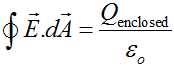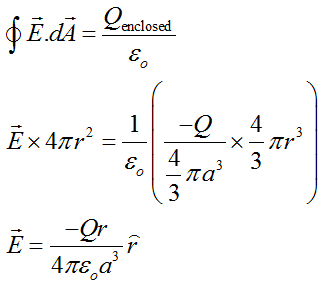# A uniformly charged ball of radius a and charge -Q is at the center of a hollow metal shell with inner radius b and outer radius c. The hollow sphere has a net charge of +2Q. Determine the strength of the electric field in the four regions: r<=a, a<r<b, b<=r<=c, r>c.

Question
38 views

A uniformly charged ball of radius a and charge -Q is at the center of a hollow metal shell with inner radius b and outer radius c. The hollow sphere has a net charge of +2Q. Determine the strength of the electric field in the four regions: r<=a, a<r<b, b<=r<=c, r>c.

check_circle

Step 1

Given:

charge on ball = -Q.

inner radius of hollow metal shell = b.

outer radius of hollow metal shell = c.

charge on hollow shell = +2Q.

Step 2

According to gauss law,where,

E = electric field.

dA = area enclosed

Qenclosed = charge enclosed in surface.

Step 3

Electric field when,

r<a.

According to gauss law,...

### Want to see the full answer?

See Solution

#### Want to see this answer and more?

Solutions are written by subject experts who are available 24/7. Questions are typically answered within 1 hour.*

See Solution
*Response times may vary by subject and question.
Tagged in